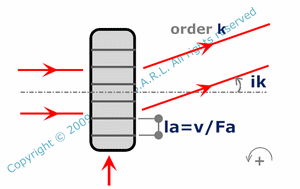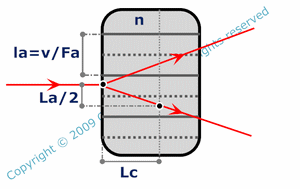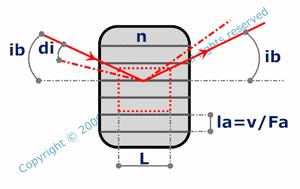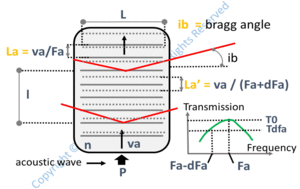and ortutorials

# acousto optic

Basics

The propagation of an elastic wave ( generated by a transducer ) in an acousto optic crystal induces periodic dilatations and compressions of the material and consequently periodic variations of its refraction index around a medium value. The crystal can be assumed to a succession of low and high index layers and may behave for light as a phase grating. In the calculations, it is assumed that the crystal is isotropic, totally transparent and that light wave has a given polarization which remains the same during its propagation. One particularity of acousto optics is that as the acoustic wave is propagating, it shifts the frequency of the diffracted light. Two situations can easily be modellized : Raman-Nath configuration and Bragg configuration. For intermediate situations analytical calculations are more complicated.

Raman Nath effectThis effect appears when the light propagates perpendicularly to the elastic wave and for short interaction lengths. The acousto optic acts then as a grating. Light wave is diffracted in different orders with different frequencies. The diffracted wave angle ( ik ) depends on the order ( k ), the wavelength ( lam ), the acoustic wave frequency ( Fa ) and its velocity ( v ).Raman Nath effect occurs for interaction lengths much smaller than a certain critical interaction length ( Lc ). Lc is the minimum distance covered by a light ray propagating perpendiculary to the acoustic wave for going through half of an acoustic wavelength ( la ) in the transverse plane ( plane perpendicular to the light wave propagation axis ). Lc is not infinite because of the natural divergence of light and the diffraction effect of the acousto optic caused by the succession of "low" and "high" index layers. Lc depends on lam, Fa, v and the medium refraction index ( n ).

Bragg conditionsIn this case, a light ray covers several "low" and "high" index layers. Interferences occur between waves diffracted by successive "low" and "high" refraction index layers. Assuming that interaction length L is much larger than Lc, a large number of layers are covered and the interferences are in general destructive. At a particular incidence angle ( corresponding to Bragg conditions ), interferences are constructive for order 1. The configuration is symmetrical. The Bragg angle ( ib ) is the angle between the incident or the diffracted light wave and the layers planes. ib depends on the wavelength ( lam ), the acoustic wave frequency ( Fa ) and its velocity ( v ).There is a tolerance of +/- di around the Bragg angle ( ib ) because the interaction length ( L ) is not infinite. This tolerance corresponds to the difference between the bragg angle ( in the air ) and the angle of incidence ( in the air ) producing an efficiency drop of 50%. di can roughly be calculated with L, the average refraction index ( n ), the elastic wave frequency ( Fa ) and its velocity ( v ).For the same reason, there is a tolerance +/- dFa on the elastic frequency Fa. Supposing that the device is working in bragg conditions for the acoustic frequency Fa, the tolerance corresponds to the difference between Fa and the frequency producing an efficiency drop of 50%. dFa can roghly be calculated with L, n, lam, v and Fa.The ratio ( T ) between diffracted power and input power depends on the acoustic power ( power of the elastic wave P ), the merit factor of the material ( M ), the wavelength ( lam ), the interaction length ( L ), the interaction height ( l ) and the average refraction index ( n ) .There is an optimal power ( Pm ) of the acoustic wave maximizing T. Pm is a function of M, lam, L, l and n.When used as a light modulator, an acousto optic crystal is crossed by a modulated elastic wave. There is a limitation to the modulation frequency mainly because of the finite velocity of the acoustic wave. The maximum modulation frequency or modulation bandwidth dF can be roughly calculated with the rise time ( t). t is the necessary time for the elastic wave to run through the beam width. t depends on the acoustic velocity and the beam width W. For a gaussian beam, the considered beam width is the beam diameter ( containing 86.5% of the light power ).

References

"Cours effets electro, acousto et magneto-optiques application à la modulation et la déflexion de la lumière" - Ecole Nationale Supérieure des Télécommunications - 1988 - author : P. Gallion.

"Notes de cours Electro-acousto optique" - Institut d'Optique Graduate School - 2009 - author : Ivan Sortais, Rémy Blandino.

"Cours études expérimentales" - Ecole Nationale Supérieure des Télécommunications - 1988 - author : P. Chabran, C. Debarge.